# Dividing unit fractions by whole numbers

##By Khan Academy

Solve a word problem by dividing a fraction by a whole number. The example used in this video is 1/5 ï¿½ï¿½ï¿½ï¿½ï¿½ï¿½ï¿½ï¿½ï¿½ï¿½ï¿½ï¿½ 4.# Recognizing fractions 1

##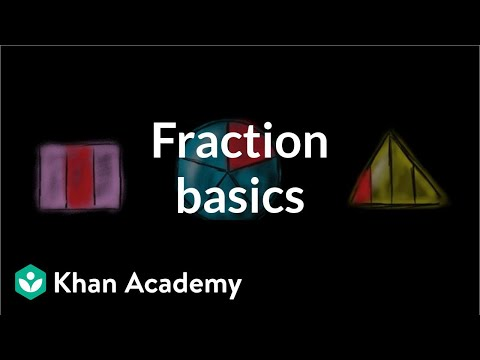By Khan Academy

Divide wholes into equal-sized pieces to create unit fractions.# That's not fair!

##By Khan Academy

Divide wholes into equal-sized pieces to create unit fractions.# Fraction basics | Fractions | 3rd grade

##By Khan Academy

Divide wholes into equal-sized pieces to create unit fractions.# That's not fair!

##By Khan Academy

Divide wholes into equal-sized pieces to create unit fractions.# Identifying unit fractions

##By Khan Academy

Divide wholes into equal-sized pieces to create unit fractions.# Dividing whole numbers by unit fractions

##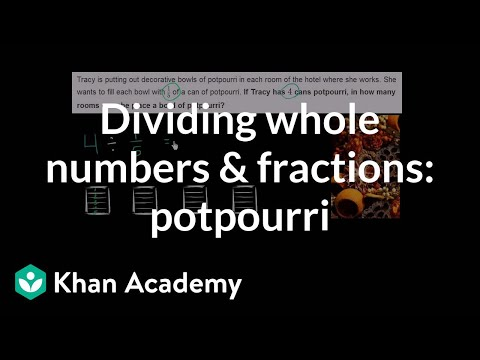By Khan Academy

Solve a word problem by dividing a whole number by a fraction. The example used in this video is 4 ï¿½ï¿½ï¿½ï¿½ï¿½ï¿½ï¿½ï¿½ï¿½ï¿½ï¿½ï¿½ 1/5.# Dividing whole numbers by unit fractions

##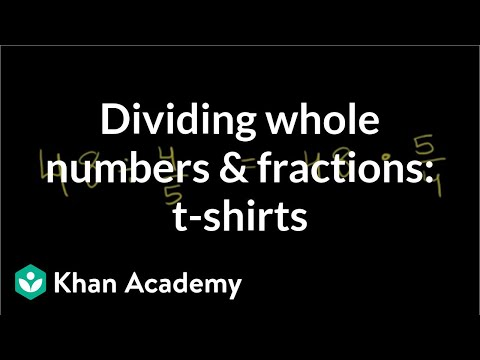By Khan Academy

Dividing a whole number by a fraction? Turn the tables and multiply by the reciprocal of the fraction. Boom!# Dividing whole numbers by unit fractions

##By Khan Academy

Dividing a whole number by a fraction? Turn the tables and multiply by the reciprocal of the fraction. Boom!# Dividing whole numbers by unit fractions

##By Khan Academy

Solve a word problem by dividing a whole number by a fraction. The example used in this video is 4 ï¿½ï¿½ï¿½ï¿½ï¿½ï¿½ï¿½ï¿½ï¿½ï¿½ï¿½ï¿½ 1/5.# Dividing whole numbers by unit fractions

##By Khan Academy

Solve a word problem by dividing a whole number by a fraction. The example used in this video is 4 ï¿½ï¿½ï¿½ï¿½ï¿½ï¿½ï¿½ï¿½ï¿½ï¿½ï¿½ï¿½ 1/5.# Dividing unit fractions by whole numbers

##By Khan Academy

Solve a word problem by dividing a fraction by a whole number. The example used in this video is 1/5 ï¿½ï¿½ï¿½ï¿½ï¿½ï¿½ï¿½ï¿½ï¿½ï¿½ï¿½ï¿½ 4.# Dividing whole numbers by unit fractions

##By Khan Academy

Dividing a whole number by a fraction? Turn the tables and multiply by the reciprocal of the fraction. Boom!# Dividing unit fractions by whole numbers

##By Khan Academy

Solve a word problem by dividing a fraction by a whole number. The example used in this video is 1/5 ï¿½ï¿½ï¿½ï¿½ï¿½ï¿½ï¿½ï¿½ï¿½ï¿½ï¿½ï¿½ 4.# Dividing whole numbers by unit fractions

##By Khan Academy

Solve a word problem by dividing a whole number by a fraction. The example used in this video is 4 ï¿½ï¿½ï¿½ï¿½ï¿½ï¿½ï¿½ï¿½ï¿½ï¿½ï¿½ï¿½ 1/5.# Dividing whole numbers by unit fractions

##By Khan Academy

Dividing a whole number by a fraction? Turn the tables and multiply by the reciprocal of the fraction. Boom!# Dividing unit fractions by whole numbers

##By Khan Academy

Solve a word problem by dividing a fraction by a whole number. The example used in this video is 1/5 ï¿½ï¿½ï¿½ï¿½ï¿½ï¿½ï¿½ï¿½ï¿½ï¿½ï¿½ï¿½ 4.# Multiplying Fractions by Whole Numbers 9-9

##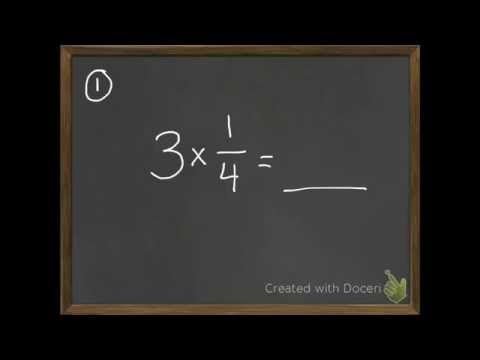By David Horan

multiply a fraction with a whole number# Multiplying Fractions by Whole Numbers 9-9

##By David Horan

multiply a fraction with a whole number.# Multiplying Fractions by Whole Numbers

##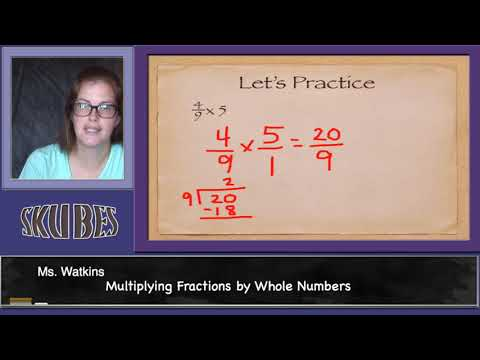By Skubes ed

Understand a multiple of a/b as a multiple of 1/b, and use this understanding to multiply a fraction by a whole number.